# DC Field Behavior

This collection of functions computes the I(V) characteristics of an individual module given the module temperature and irradiance. The tool uses the 1-diode model. At this point in the simulation process, the irradiance seen by the PV module has been corrected for all optical losses.

# Module Temperature

Two models for estimating module temperature are supported by PlantPredict and selectable by the user in the Simulation Settings: the Sandia method and the static heat-balance model.

# Sandia Module Temperature

This routine empirically computes the module temperature from the air temperature, the wind speed, and the in-plane irradiation. This model uses two empirically-determined coefficients: at establishes the upper limit for module temperature at low wind speeds and high solar irradiance, bt establishes the rate at which module temperature drops as wind speed increases.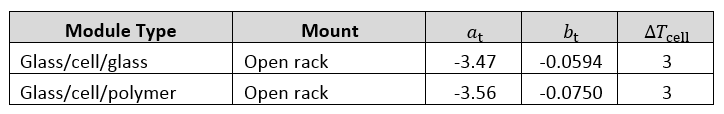## Inputs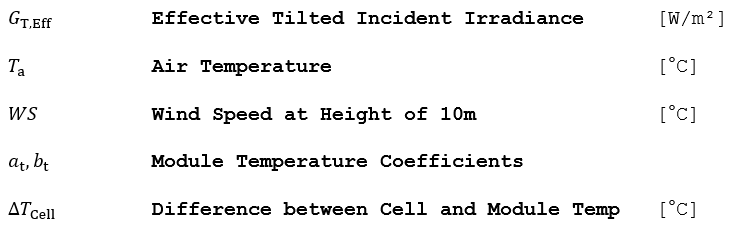## Outputs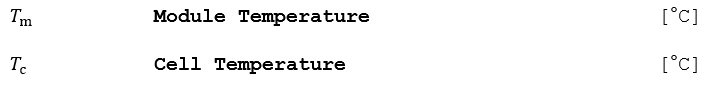1.) Compute the module temperature as a function of the available insolation, wind speed, and air temperature: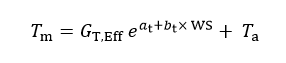2.) Compute the cell temperature. This temperature difference is typically 2 to 3 °C for flat-plate modules in an open-rack mount at a reference irradiance GRef of 1000 W/m2.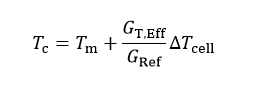## Reference

King, D. L., Kratochvil, J.A., Boyson, W.L., Photovoltaic Array Performance Model, Report SAND2004-3535, Sandia National Laboratories, Albuquerque, NM, September 1997.

# Heat Balance Temperature Model

The thermal behavior of the field, which strongly influences the electrical performances, is determined by a thermal balance between the ambient temperature and the cell’s temperature, which is elevated by incident irradiation. In this simple model, αT is the absorption coefficient of solar irradiation, and ηm is the PV efficiency (related to the Module area), i.e. the removed energy from the module.

## Inputs## Outputs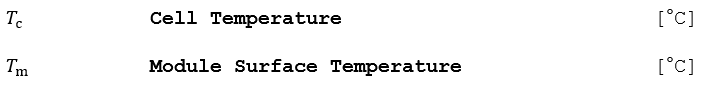## Algorithm

1.) Compute the module temperature as a function of the available insolation, wind speed, and air temperature: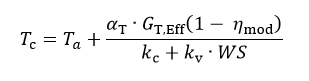The usual value of the absorption coefficient α is 0.9. The PV efficiency is taken from the Module File.

The thermal behavior is characterized by a thermal loss factor designated here by k, which can be split into a constant component kc and a factor proportional to the wind velocity kv. These factors depend on the mounting mode of the modules (sheds, roofing, facade, etc.).

2.) Convert the cell temperature to a module surface temperature.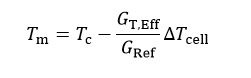## Reference

Mermoud, A., Conception et dimensionnement de systèmes photovoltaïques : Introduction des modules PV en couches minces dans le Logiciel PVsyst. Université de Genève, 2005.

# Module I(V) Curve

A complete module I(V) curve is computed under ambient conditions for the current time step. The “One-Diode” parameters are defined in the module file. The one-diode model uses an equivalent electrical circuit model and the governing diode equation to generate an explicit, continuous current vs. voltage function.

# One-Diode Model

Given the 1-diode model parameters for the module, this routine computes the module’s current-voltage characteristics. Figure 35 shows the equivalent circuit of a PV cell. The following algorithm includes the recombination current, Irec, a refinement in the model that can be set to zero if the parameters required to determine the current (μ, τeff, di, Vbi) are not provided in the module definition input file.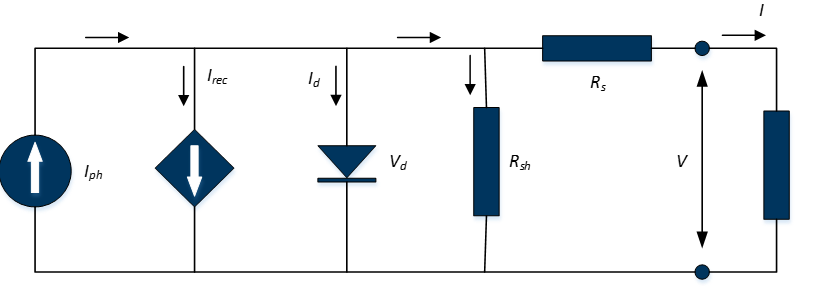## Inputs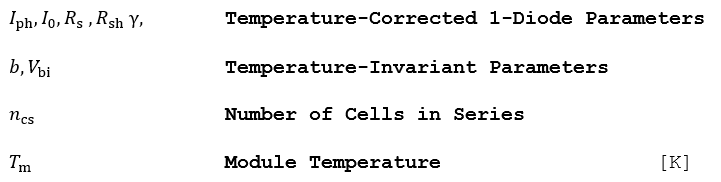## Outputs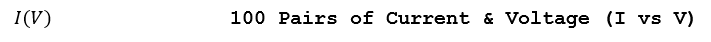## Algorithm

1.) Solve the following transcendental equation that relates the module’s output current i and output voltage υ to the photocurrent Iph, and the 1-diode parameters corrected for the temperature and irradiance level (I0, Rsh, Rs, γ).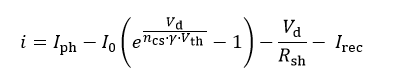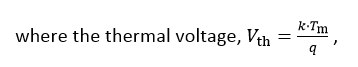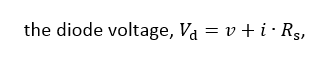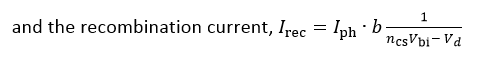The parameter b, given in the module parameter definition, combines the following terms: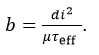Expanded, the transcendental function for the output current becomes: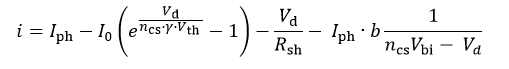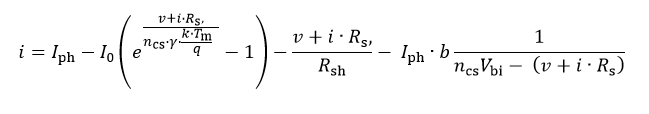2.) The current should be solved for diode voltages in the interval [0, νoc,g], where νoc,g is the guess for the open-circuit voltage, and can be found by setting the I to zero in the above equation (neglecting Irec):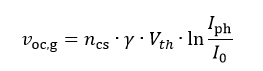3.) The limits of the I(V) curve in the first quadrant are defined by the open-circuit voltage and short-circuit current, which can be found by setting i and ν to 0, respectively.

4.) Find the maximum power point diode voltage using the Newton-Raphson method by iteratively solving the following equation until the difference between Vd,n+1 and νd,n is arbitrarily small.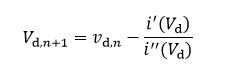5.) Find the maximum power point current by solving the 1-diode equation.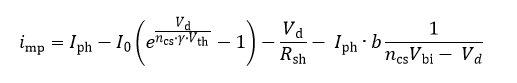6.) Find the maximum power point voltage at the output terminal of the equivalent circuit by subtracting the voltage drop across the series resistance.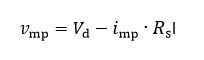## Reference

Mermoud, A., Conception et dimensionnement de systèmes photovoltaïques : Introduction des modules PV en couches minces dans le Logiciel PVsyst. Université de Genève, 2005.

# One-Diode Model Temperature Correction

Given the 1-diode model parameters defined for the module under STC conditions (25 °C, 1000 W/m2), compute the temperature and irradiance-corrected parameters at the actual module temperature Tm and the available solar energy GT,Eff. The default temperature correction of all 1-diode parameters is linear. If a non-linear temperature correction of the diode ideality factor is desired, the then a set of additional parameters (polynomial coefficients) is available to affect this correction.

This non-linear response is illustrated in Figure 36 for a family of I(V) curves with a module temperature range of 8 °C to 75 °C. This data was measured in the laboratory using the module temperature control system and a Spire long-pulse solar simulator.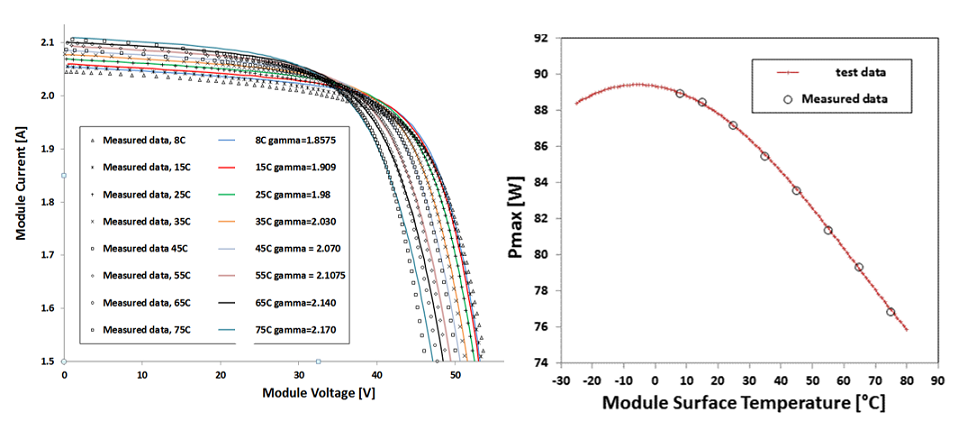## Inputs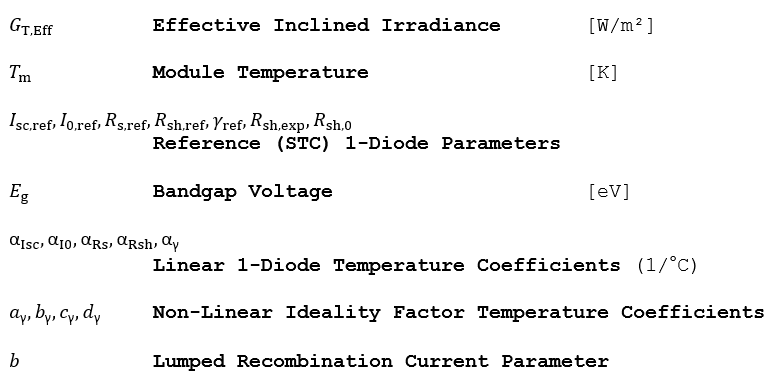## Outputs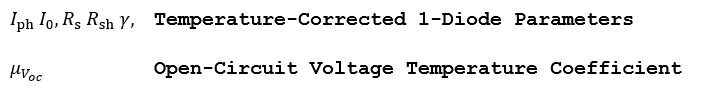## Algorithm

1.) Given the effective available insolation GT,Eff and module temperature Tm, find the corrected 1-diode shunt and series resistance.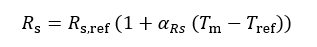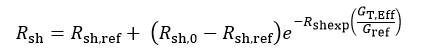2.) If the linear correction to the diode ideality factor is desired, or all non-linear coefficients ay,by,cy,dy = 0, then correct it for the module temperature as follows: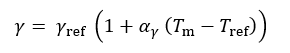3.) If the non-linear correction to the diode ideality factor is desired, then correct it for the module temperature as follows: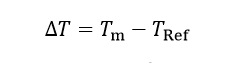4.) Correct the saturation current for temperature. Note that this correction includes the previously temperature-corrected diode ideality factor.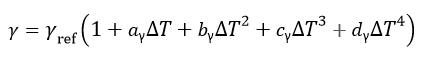*  In the above equation aγ is referring to the y1 non-linear temperature coefficient in PlantPredict and bγ is referring to y2, cγ is referring to y3 and dγ is referring to y45.) Find the short-circuit current under the ambient conditions.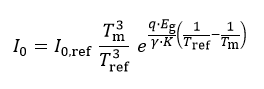6.) Even when a module is biased in short-circuit condition, a voltage drop occurs across Rs. As a result, the junction still experiences a voltage bias and there are currents flowing through both the recombination path and through Rsh when the terminal voltage of the device is zero, i.e. the recombination term does not go to zero in the short circuit condition. Therefore the photocurrent, Iph must be greater than Isc in order to supply parasitic currents as well as the external current which has the rated ISC,ref value. Find the actual Iph as follows: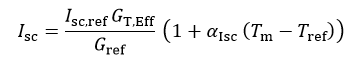The temperature dependence on voltage is also computed, but not used elsewhere in the simulation. Note that μΙsc ≅ αIph, given in 1/°C.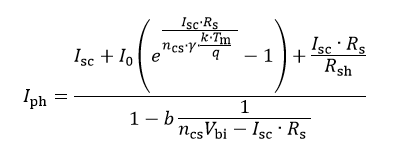## Reference

Schwieger, M., Michalksi, S., Non-Linearity of Temperature Coefficients, Equivalent Cell Temperature and Temperature Behaviour of Different PV-Module Technologies. TÜV Rheinland Energie und Umwelt, Cologne, Germany. Proceedings from the 28th EU PVSEC.

# Mismatch

Compute the power lost due to module I(V) curve characteristic mismatches within one module bin. This is an empirical constant and suppresses the maximum power point on the I(V) curve. The mismatch can be applied to voltages within the vicinity of the I(V) curve’s knee, but is undefined for the array’s Isc and Voc.

## Inputs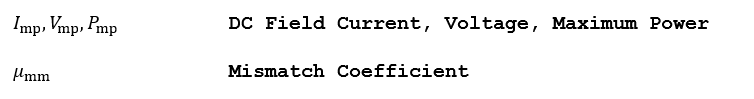## Outputs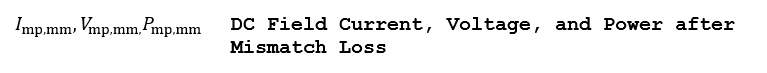## Algorithm (Provisional)

1.) The mismatch factor is applied directly to the effective irradiance, prior to the evaluation of the 1-diode or Sandia models: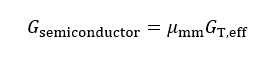## Algorithm (Future)

Mismatch may in future be embedded as part of a DC degradation algorithm. A simple solution can include the following.

1.) The depressed maximum power point is found as follows: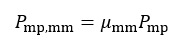2.) To first order, the mismatch’s contribution to the current and voltage is weighted equally: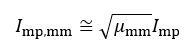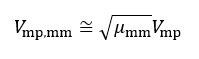# DC Field I(V) Curve

This routine aggregates the I(V) curves from individual modules, and then sums them in parallel and in series to yield an I(V) curve profile that is representative of the entire DC Field. Figure 37 shows a general schematic of such parallel-series connection.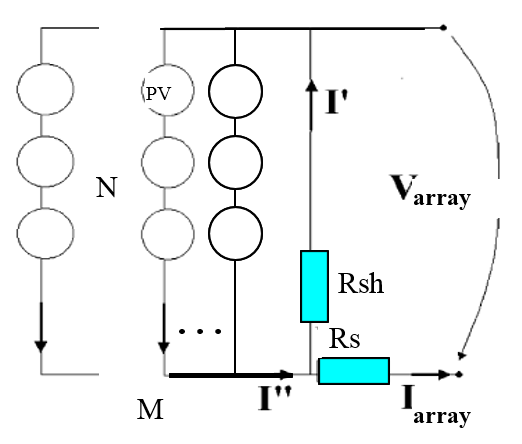## Inputs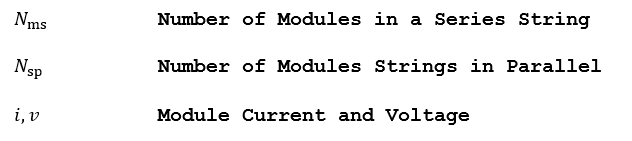## Outputs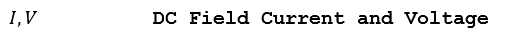## Algorithm

1.) For combination of identical modules in series and parallel, the points on the I(V) curves or current and voltages can be scaled as follows:

a.) Compute the aggregate I(V) curve for a series string comprised of Nms identical modules. The voltages can be multiplied by the number of modules in the series string; the current remains unchanged: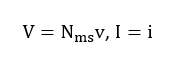b.) Compute the I(V) curve for a parallel connection of Nsp identical series strings in parallel modules. The currents can be multiplied by the number of strings in parallel; the voltage remains unchanged: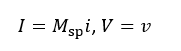2.) For heterogeneous arrays comprised of modules that do not exhibit the same I(V) curve (i.e. a cool shaded module connected to a hot unshaded module), the individual I(V) curves must be added:

a.) Parallel connection: Combine heterogeneous modules or strings connected in parallel by interpolating the I(V) array at common voltage points so that the sampled currents are aligned; then sum the individual currents.

b.) Series connection: Combine heterogeneous modules or strings connected in series by interpolating the I(V) array at common current points so that the sampled voltages are aligned; then sum the individual voltages.

# DC Wiring

This routine computes the power lost due to Joule heating losses within the DC portion of the array. The resulting current and voltage is that seen at the inverter terminals. The resistance can also be estimated if a percent Joule loss (PJL) at STC is provided instead of the equivalent bulk resistance. The resistance from a percent Joule loss is estimated as follows from the module’s rated STC current and voltage and number of series & parallel connections in the DC Field: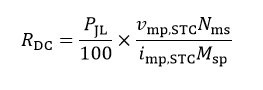## Inputs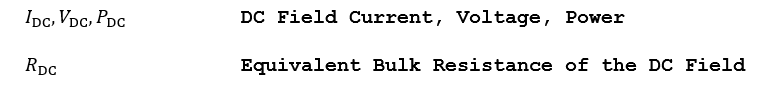## Outputs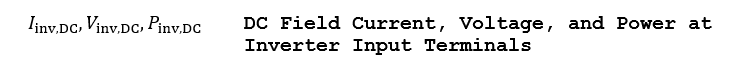## Algorithm (Delta Series Resistance)

1.) Given an equivalent bulk resistance RDC, find the delta series resistance seen by one module as follows: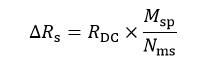2.) This ΔRS is then added to the series resistance in the one-diode model.

3.) An approximation of the power dissipated by the bulk resistance can be obtained from the DC Field power seen by the inverter is as follows: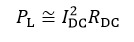# DC Health

This factor is a Catch-All loss factor to account for common problems in utility-scale powerplants. These can be blown fuses, faulty connections, broken modules, bird droppings or shadings from plants and the like. Typically these losses are lost in the usual data noise. The default value for this loss is 1%.

# Degradation and Plant Construction Schedule

Large power plants are not constructed and commissioned at one point in time, but come on line on a Block-by-Block basis. Therefore, different sections of the plant will start contributing energy staggered in time as each comes on line, and accounting for energization times lasts until the last section is energized and the full capacity of the plant is realized.

In addition, the long term power plant production reduces continuously over time due to environmental exposure, component aging, and accrued module defects. Three mutually-exclusive models are supported, and can be selected by the user on simulation setup: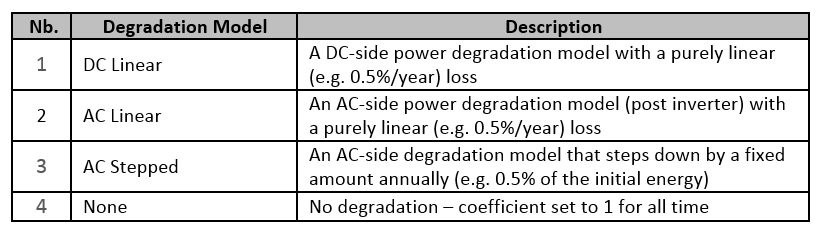### Figure 38. List of Degradation Models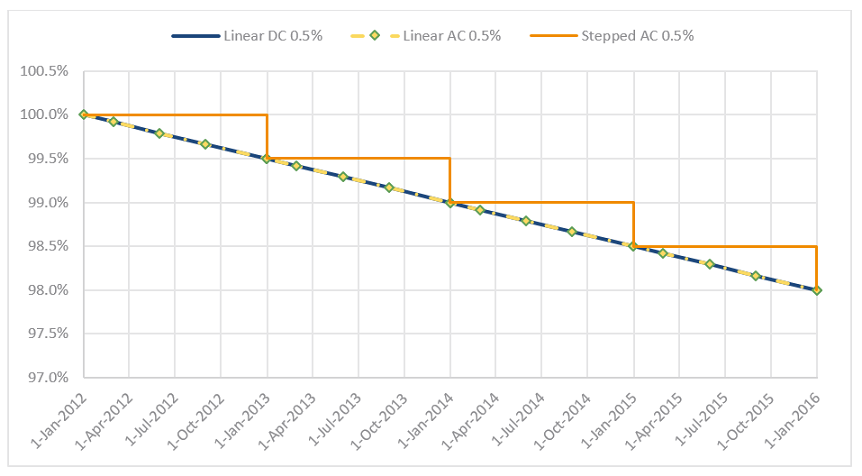# DC Linear

Linear DC degradation function reduces the DC power input to the inverter as a linear function of time by the specified rate from the user.

## Inputs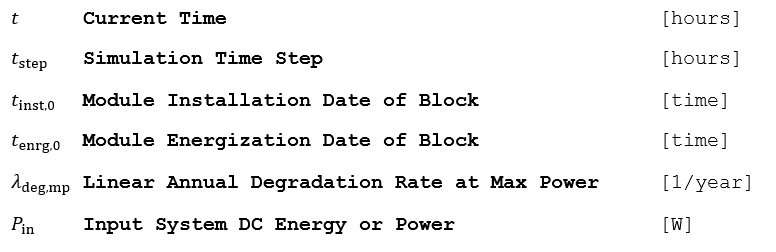## Outputs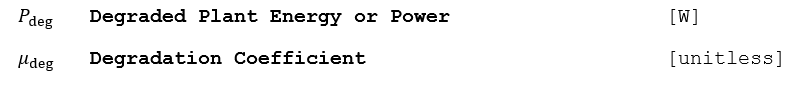## Algorithm

1.) Loop for all simulation steps for each Block

a.) The continuous-time function in hours is as follows: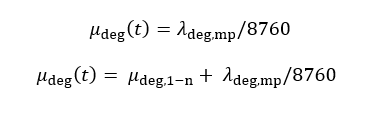b.) Find the system power due to energization time and degradation for time t.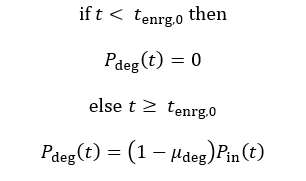# AC Linear

This function will reduce the AC power output as a linear function of time.

## Inputs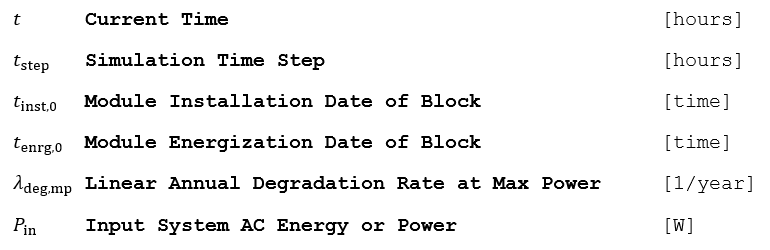## Outputs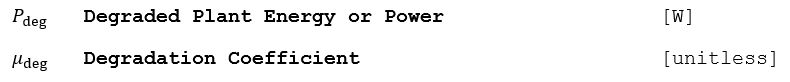## Algorithm

1.) Loop for all simulation steps for each Block.

a.) The continuous-time function in hours is as follows:b.) Find the system power due to energization time and degradation for time t.# AC Stepped

This function will reduce the AC power output as a stepped function of time. The AC output of the block is reduced by the linear annual degradation rate at a discrete point in time.

## Inputs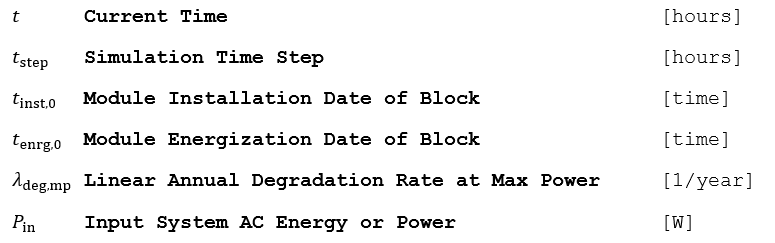## Outputs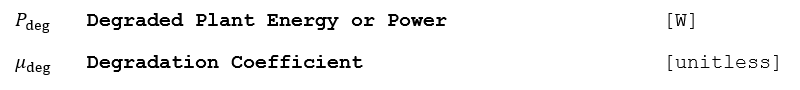## Algorithm

1.) Loop for all simulation steps for each Block

a.) The discrete-time function in hours is as follows: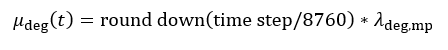b.) Find the system power due to energization time and degradation for time t.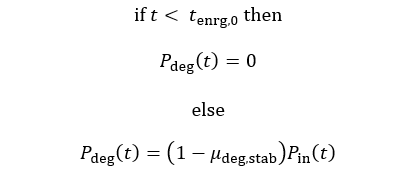# Light and elevated Temperature Induced Degradation (LeTID)

Light and elevated temperature induced degradation (LeTID) is another forms of power output degradation that affects crystalline silicon (c-Si) solar cell technology.

This degradation effect can be seen in both an immediate drop in module power and a sustained degradation rate over many years. LeTID is present in both p-type and n-type silicon cells and universally affects today’s most widely used c-Si module types.

LeTID occurs on a longer time scale on the order of multiple years. This blind spot requires additional treatment in the energy prediction to ensure an accurate performance prediction based on best available data for a given module technology type, specific manufacturer and cell process. The algorithm used is the same as in DC Linear degradation.

If manufacturer or independent test data is obtained, it should serve as a basis for incorporated loss factors. In absence of this module specific data, the table below summarizes the default settings for the energy prediction loss factors in Plant Predict for each module technology type based on the studies surveyed and referenced below.

Category Parameter Value or Setting
P-Type c-Si,
MonoPERC,
Cast-Mono
(Mono and Bifacial)
N-Type c-Si,
Ga Doped P-Type
(Mono and Bifacial)
LeTID First Year LeTID degradation rate 1% 0.5%